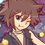# Geometry (Thailand Math POSN 3rd round)

Theorems allowed to use: Basic theorems, Isogonal conjugate, Symmedian point, Gergonne point, Nagel point, Adam Circle, Tucker Circle, Tucker Hexagon, Taylor Circle.

Gergonne point: 3 lines that pass through the vertex and the contact point of incircle are concurrent at Gergonne point.

Nagel point: 3 lines that pass through the vertex and the contact point of excircle are concurrent at Nagel point.

Tucker Circle: see Tucker Circle

Taylor Circle: see Taylor Circle

Write a full solution.

1. Prove that Gergonne point of $\triangle ABC$ is a symmedian (Lemoine point) of Gergonne triangle.

2. Prove that 3 lines that pass through excenters of $\triangle ABC$ and midpoints of sides of $\triangle ABC$ that are closest to excenters are concurrent.

3. Let $\triangle DEF$ and point $G$ be Gergonne triangle and Gergonne point of $\triangle ABC$, and $D,E,F$ are opposite to $A,B,C$.

4. Let $T$ be a center of Taylor circle in $\triangle ABC$. Prove that $AT^{2} - h_{a}^{2} = BT^{2}-h_{b}^{2} = CT^{2}-h_{c}^{2}$ where $h_{a},h_{b},h_{c}$ are altitudes of $\triangle ABC$ from vertices $A,B,C$ respectively.

This note is part of Thailand Math POSN 3rd round 2015Note by Samuraiwarm Tsunayoshi
6 years, 3 months ago

This discussion board is a place to discuss our Daily Challenges and the math and science related to those challenges. Explanations are more than just a solution — they should explain the steps and thinking strategies that you used to obtain the solution. Comments should further the discussion of math and science.

When posting on Brilliant:

• Use the emojis to react to an explanation, whether you're congratulating a job well done , or just really confused .
• Ask specific questions about the challenge or the steps in somebody's explanation. Well-posed questions can add a lot to the discussion, but posting "I don't understand!" doesn't help anyone.
• Try to contribute something new to the discussion, whether it is an extension, generalization or other idea related to the challenge.

MarkdownAppears as
*italics* or _italics_ italics
**bold** or __bold__ bold
- bulleted- list
• bulleted
• list
1. numbered2. list
1. numbered
2. list
Note: you must add a full line of space before and after lists for them to show up correctly
paragraph 1paragraph 2

paragraph 1

paragraph 2

[example link](https://brilliant.org)example link
> This is a quote
This is a quote
    # I indented these lines
# 4 spaces, and now they show
# up as a code block.

print "hello world"
# I indented these lines
# 4 spaces, and now they show
# up as a code block.

print "hello world"
MathAppears as
Remember to wrap math in $$ ... $$ or $ ... $ to ensure proper formatting.
2 \times 3 $2 \times 3$
2^{34} $2^{34}$
a_{i-1} $a_{i-1}$
\frac{2}{3} $\frac{2}{3}$
\sqrt{2} $\sqrt{2}$
\sum_{i=1}^3 $\sum_{i=1}^3$
\sin \theta $\sin \theta$
\boxed{123} $\boxed{123}$